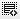## What is the table_array argument?0
array arqument means why it s used?0

A table array is one of the arguments used in Excel's lookup functions, such as VLOOKUP and HLOOKUP.

The LOOKUP functions search the table array to find specific information.

For VLOOKUP (vertical lookup), the table_array must contain at least two columns of data.

For HLOOKUP (horizontal lookup), the table_array must contain at least two rows of data.

Other arguments in the LOOKUP functions that make use of the table array:

lookup _value: located in the first column or row of the table_array.0

A table array is one of the arguments used in Excel's lookup functions, such as VLOOKUP and HLOOKUP.

The LOOKUP functions search the table array to find specific information.

For VLOOKUP (vertical lookup), the table_array must contain at least two columns of data.

For HLOOKUP (horizontal lookup), the table_array must contain at least two rows of data.

Other arguments in the LOOKUP functions that make use of the table array:

lookup _value: located in the first column or row of the table_array.

index_num: The number of the column or row in the table_array that contains the data you are searching for.

Click on thisicon to add code snippet.